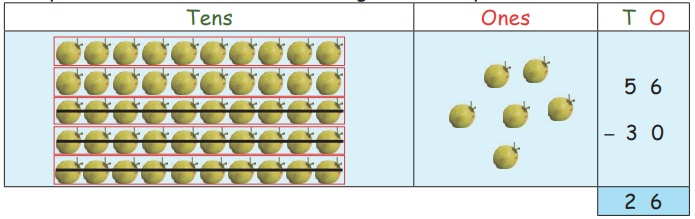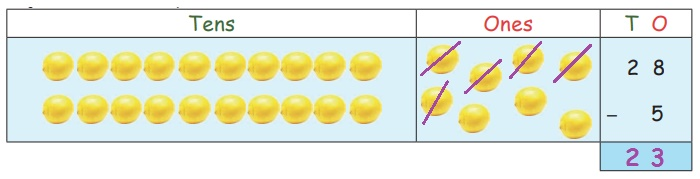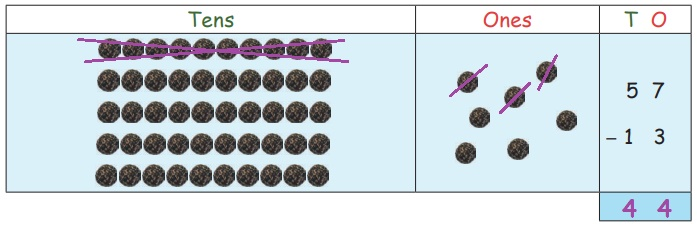Home | | Maths 2nd Std | Subtraction

# Subtraction

Teacher’s Note: Make the children to understand the difference between the numbers and help to find answer.

Subtraction

Recall

Observe the picture and write the subtraction fact by striking out the objects.

1. Write the subtraction fact inside the box to get the difference 2. One is done for you.2. Write the number statement to get the answer 6.3. Write the subtraction fact to get the answer 1.4. Subtract the following.Teacher’s Note: Make the children to understand the difference between the numbers and help to find answer.

Travel Through

Birthday celebration

Satish distributed 20 peanut cakes (kadalai mittai) to his friends on his birthday.Complete the subtraction fact.20 – 4 = 16

16 – 3 = 13

13 – 5 = 8

8 – 2 = 6

6 – 3 = 3

3 – 3 = 0Keywords: Take away, Difference, Remaining, Left, Balance

Teacher’s Note: Teacher can create more subtraction facts from the above situation by varying the number of peanut cakes taken by the children.

Learn

1. Kamali had 25 cucumbers She distributed 3 of them to her friends. How many cucumbers are left with kamali?2. There are 56 tender coconuts in a shop 30 are sold out. How many tender coconuts are remaining in the shop?Practice

1. Leela plucked 28 lemons and used 5 lemons for preparing juice. How many lemons were left?28 − 5 = 23

Ans : 23

2. Bala made 26 paper boats and Kala made 6 paper boats. How many paper boats did Bala make more than Kala?26 − 6 = 20

Ans : 20

3. Siva had 36 peanuts, He ate 14 peanuts in the play time. How many peanuts remain in his pocket?36 ­ − 14 = 22

Ans : 22

4. Kaviya’s mother prepared 57 sesame balls. She ate 13 sesame balls with her friends. How many sesame balls are left with her?57 − 13 =  44

Ans : 44

Learn

Various ways of subtraction

1. Subtraction using fingers : 38  52. Subtraction using number line 38 – 538 − 25 =13

Subtraction using beads 38 – 2538 – 25 = 13

Subtract using abacus 98 – 259 8 – 2 5 = 7 3

Practice

i. Subtract by striking the beads

48 – 5Ans : 43

78 – 35Ans : 43

Subtract using abacus

36 – 3 = 33Ans : 33

69 – 47 = 22Ans : 22

ii. Find the value.Know More

For any given addition fact, we can form 2 subtraction facts.Activity

* Teacher shall divide the class into groups of two members and place 9 bundles of 10 sticks in a box and 9 single sticks in a box.

* Teacher shall write a subtraction fact on the board and call one group to find the answers.

* One member should pick up the bundle of tens and other member should pick up the loose sticks according to the number.

* They shall subtract the numbers by putting the bundles and loose sticks back in box and then show the bundles and loose sticks left with them.

* Teacher can continue the activity with other children.

Think like a Mathematician

Observe the patterns and complete the subtraction facts.Ans : 55, 31, 21, 10, 5

Pleasure Time

Complete the outer circle by writing the subtraction fact. One is done for you.Try This

a. Find the answer for the subtraction facts given below.

37 – 30 = 7

46 – 41 = 5

68 – 54 = 14

70 – 70 = 0

57 – 42 = 15

21 – 0 = 21

39 – 20 = 19

b. Create more subtraction facts using the following numbers 0, 20, 21, 30, 37, 39, 41, 42, 46, 54, 57, 68, 70 and find the solution.

0 – 20 = 20

30 – 21 = 9

39 – 37 = 2

46 – 39 = 7

42 – 41 = 1

57 – 54 = 3

70 – 68 = 2

Mental Maths

1. Ilakiya made a bouquet with 29 flowers. As she was arranging the bouquet, 12 flowers fell off. How many flowers were left in the bouquet?

Ans :29 – 12 =  17

2. There are 19 children in the park. 2 of them went out of the park. How many children are inside the park?

Ans :19 – 2 = 17

3. There were 33 birds in the garden. 11 flew away. How many birds were left in the garden?

Ans :33 – 11 = 22

4. There are 64 goats in a farm. 11 of  them were taken to another farm. How many goats are left?

Ans :64 – 11 = 53

5. There are 38 paint brushes in the box. 10 brushes are used. How many brushes are unused?

Ans :38 – 10 = 28

Tags : Numbers | Term 1 Chapter 2 | 2nd Maths , 2nd Maths : Term 1 Unit 2 : Numbers
Study Material, Lecturing Notes, Assignment, Reference, Wiki description explanation, brief detail
2nd Maths : Term 1 Unit 2 : Numbers : Subtraction | Numbers | Term 1 Chapter 2 | 2nd Maths